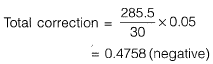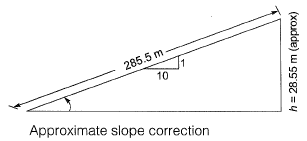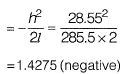Courses

# Fundamental Concepts of Surveying and Linear Measurement Civil Engineering (CE) Notes | EduRev

## Civil Engineering (CE) : Fundamental Concepts of Surveying and Linear Measurement Civil Engineering (CE) Notes | EduRev

The document Fundamental Concepts of Surveying and Linear Measurement Civil Engineering (CE) Notes | EduRev is a part of the Civil Engineering (CE) Course Topic wise GATE Past Year Papers for Civil Engineering.
All you need of Civil Engineering (CE) at this link: Civil Engineering (CE)

Question 1: A survey line was measured to be 285.5 m with a tape having a nominal length of 30 m. On checking, the time length of the tape was found to be 0.05 m too short. If the line lay on a slope of 1 in 10. the reduced length (horizontal length) of the line for plotting of survey work would be    [2019 : 2 Marks, Set-I]
(a) 285.6 m
(b) 284.5 m
(c) 283.6 m
(d) 285.0 m

Answer: (c)
Solution: Given:
Measured length = 285.5 m
Designated tape length = 30 m
Actual tape length = 30 - 0.05 = 29.95 m
Slope along measurement = 1 in 10.
1. Standardization correction,
Actual tape length l' < l (hence negative correction)
Correction per tape length
= 29.95 - 30 = -0.05 m2. Slope correction:Total correction=-(1.4275 + 0.4758)
= -1.9033
Correct length = Measured length - Correction
= 285.5 - 1.9033
= 283.596
= 283.6 m

Question 2: The accuracy of an Electronic Distance Measuring Instrument (EDMI) is specified as ± (a mm + b ppm). Which one of the following statements is correct?    [2017 : 1 Mark, Set-I]
(a) Both a and b remain constant, irrespective of the distance being measured.
(b) a remains constant and b varies in proportion to the distance being measured.
(c) a varies in proportion to the distance being measured and b remains constant.
(d) Both a and b vary in proportion to the distance being measured.
Answer: (b)
Solution: Accuracy of EDMI is generally stated in terms of constant instruments error and a measuring error proportional to the distance being measured: ± (a mm + b ppm). The first part indicates a constant instrument error that is independent of the length of the line measured. The second component is the distance related error.

Question 3: The survey carried out to delineate natural features, such as hills, rivers, forests and man-made features, such as towns, villages, buildings, roads, transmission lines and canals is classified as    [2014 : 1 Mark, Set-II]
(a) engineering survey
(b) geological survey
(c) land survey
(d) topographic survey
Answer: (d)
Solution: Typographic survey is done to determine the natural features of a country such as rivers, strains, lakes, wood, hills etc. and artificial features such as roads, railways, canals, towns and villages.

Offer running on EduRev: Apply code STAYHOME200 to get INR 200 off on our premium plan EduRev Infinity!

69 docs

,

,

,

,

,

,

,

,

,

,

,

,

,

,

,

,

,

,

,

,

,

;